# PSAT Math : Exponential Ratios and Rational Numbers

## Example Questions

← Previous 1

### Example Question #531 : Algebra

Ifandare positive integers and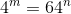, then what is the value of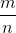?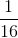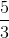Explanation:

43 = 64

Alternatively written, this is 4(4)(4) = 64 or 43 = 641.

Thus, m = 3 and n = 1.

m/n = 3/1 = 3.

### Example Question #1 : How To Find A Ratio Of Exponents

Write the following logarithm in expanded form: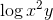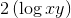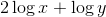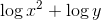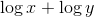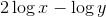Explanation: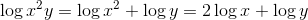### Example Question #231 : Exponents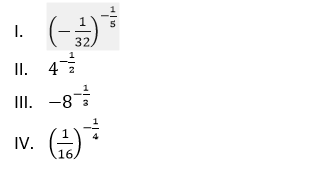Which of the following lists the above quantities from least to greatest?

II, III, I, IV

I, III, II, IV

IV, III, II, I

I, IV, II, III

I, IV, III, II

I, III, II, IV

Explanation: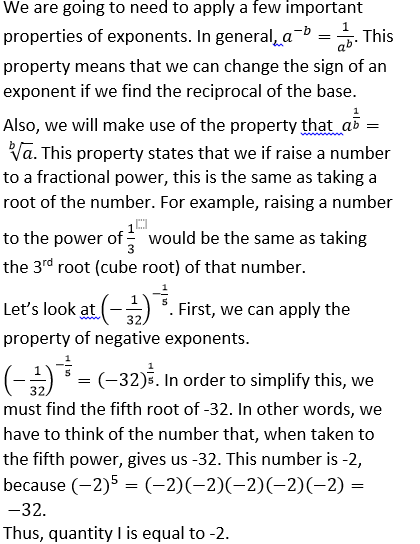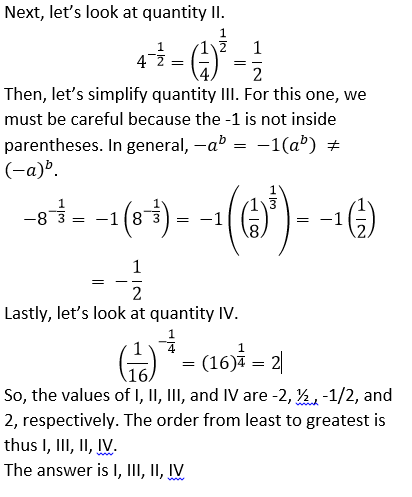### Example Question #1 : Exponents And Rational Numbers

Solve for.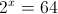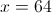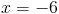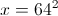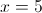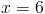Explanation:

Since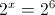Hence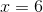### Example Question #2 : Exponents And Rational Numbers

Simplify: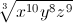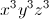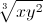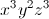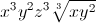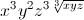Explanation: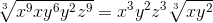### Example Question #3 : Exponents And Rational Numbers

Solve for: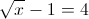Explanation:

From the equation one can see that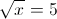Hencemust be equal to 25.

### Example Question #4 : Exponents And Rational Numbers

Evaluate: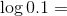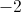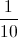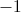Explanation: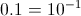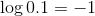### Example Question #5 : Exponents And Rational Numbers

Solve for.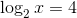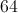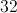Explanation: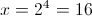### Example Question #1 : Exponents And Rational Numbers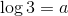and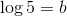Find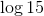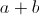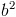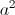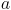Explanation: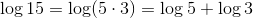Hence the correct answer is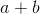.

### Example Question #1 : How To Find An Exponent From A Rational Number

Solve for.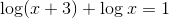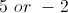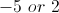Explanation:

Use the rules of logarithms to combine terms.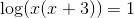Hence,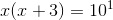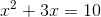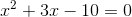By fatoring we get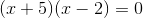Hence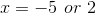.

However, you cannot take the logarithm of a negative number. Thus, the only value foris.

← Previous 1